8-120.

Missing problem for Book CCA2 => Chapter Ch8 => Lesson 8.3.1 => Problem 8-120

Created from orphaned homework help problem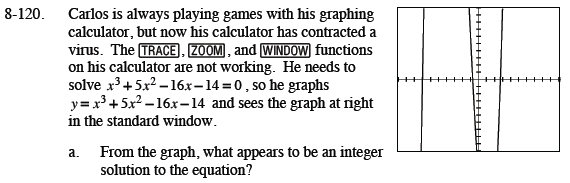x = -7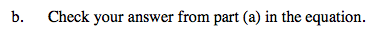Substitute your answer from part (a) into the original equation.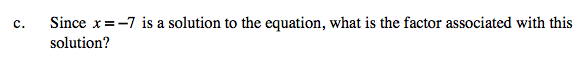(x + 7)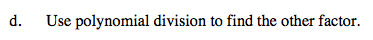Divide x³ + 5x² − 16x − 14 by x + 7
using a generic rectangle.

x2 − 2x − 2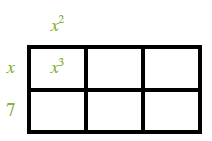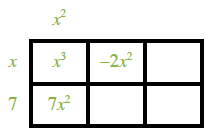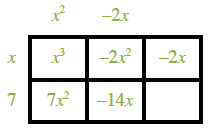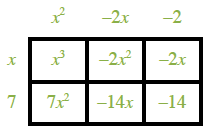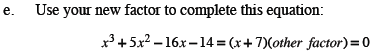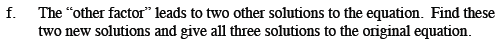Use the Quadratic Formula with the answer found in part (d) that you used for part (e).